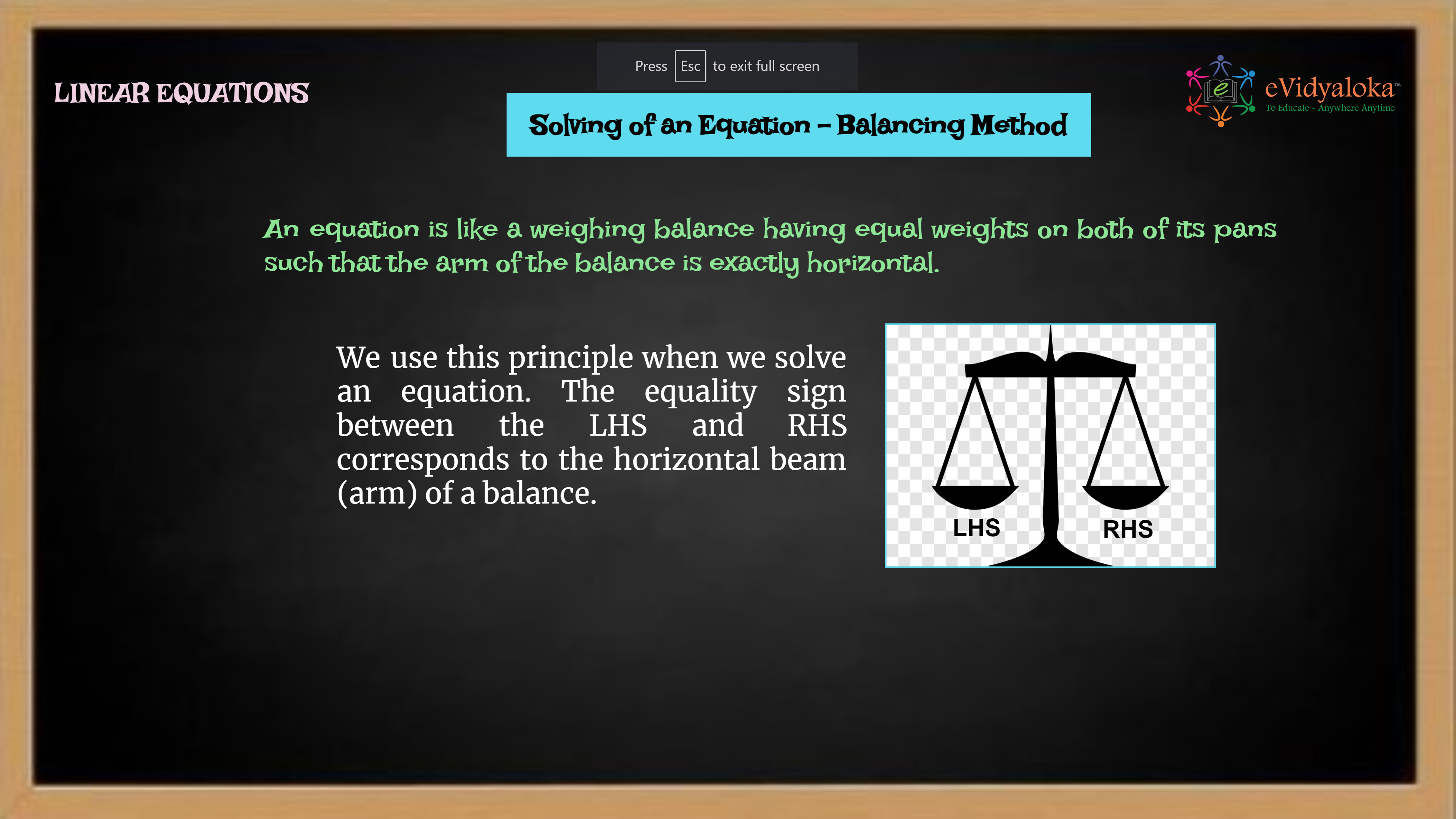# Solving Linear Equations

``````

Dear Volunteer Teacher,

Kindly teach the bridge concept of Solving Linear Equations prior to teaching the related textbook chapters.
Thank you.

## Course Content

• Introduction of equation
• Equality and inequality
• Setting an equation
• Solving an equation by balancing method
• Solving an equation by transpose method
• Difference ways to express an equation
• Ways to provide practical solution by applying equation

## Learning Outcomes

Learning outcomes has been listed below which will serve as your guide for what students need to learn.

SWBAT:

• define an equation and understand the solutions to it.
• set up an equation and solve it by Balancing and Transposing methods.
• understand the Solution to an Equation - reversal process.

## PPT Deck

To make the lesson come alive, please find below PPT deck